##### Question

In: Statistics and Probability

# Mr. Cooper recently gave a test to his 50 student history class. The scores were normally...

Mr. Cooper recently gave a test to his 50 student history class. The scores were normally distributed with a mean of 75 and a standard deviation of 7.

1) What percentage of students scored higher than​ 85?​% (Round to two decimal​ places)

2) What percentage of students scored between 73 and​ 80?% ​(round to two decimal​ places)

3) 75% of the students scored higher than what​ score? ​(Round to two decimal​ places)

​4) 30% of students scored lower than what​ score? (Round to two decimal​ places)

5) Approximately how many students got a C​ (70) or higher on the​ exam?  nothing

6) Approximately how many students got an A​ (90+) on the​ exam?

## Solutions

##### Expert Solution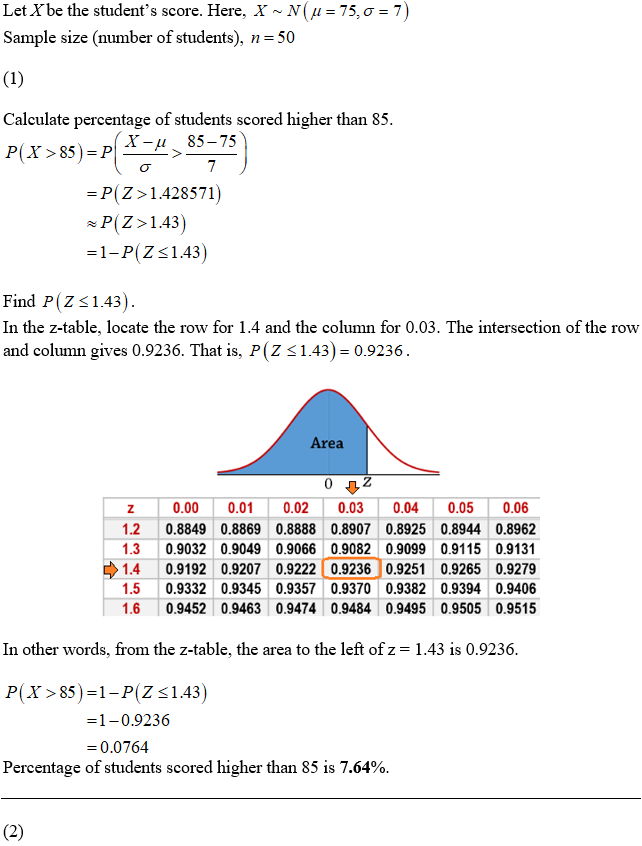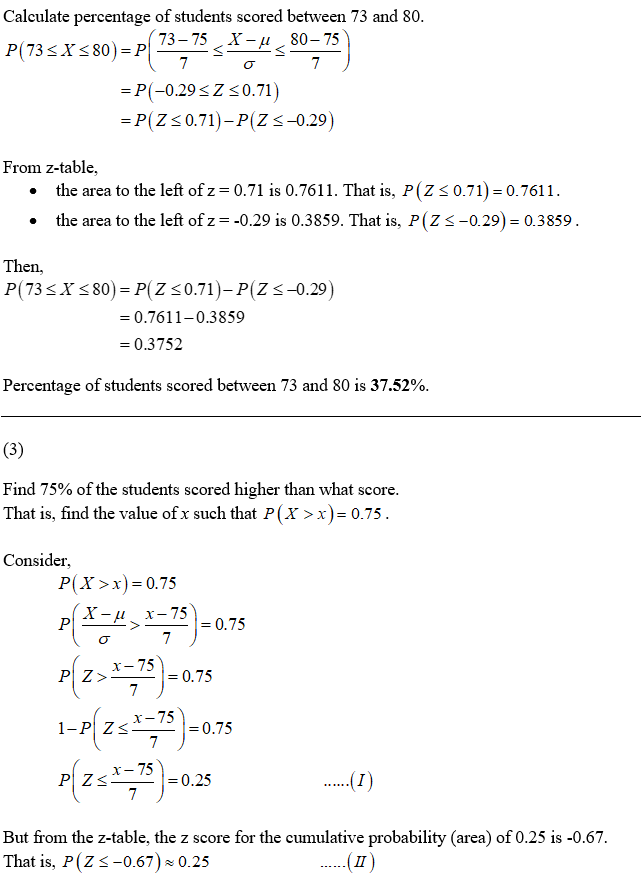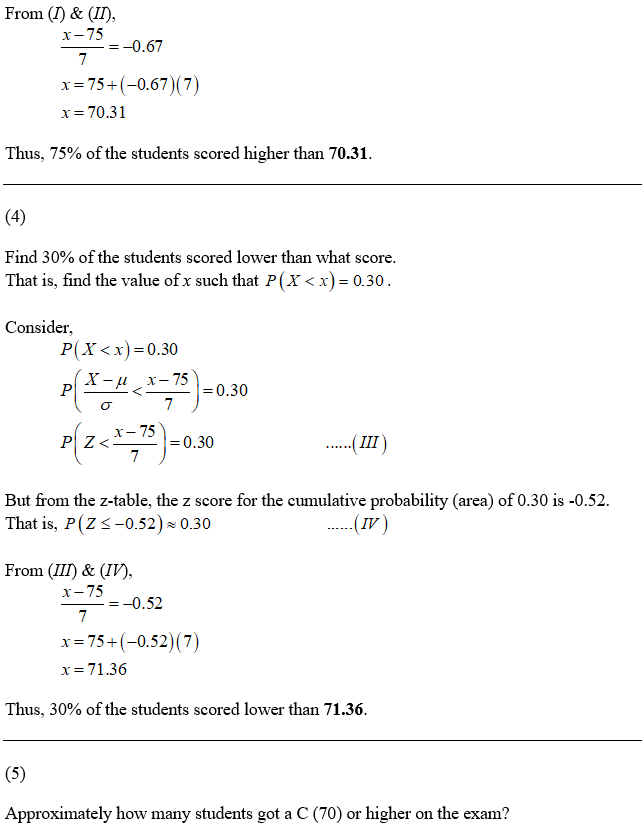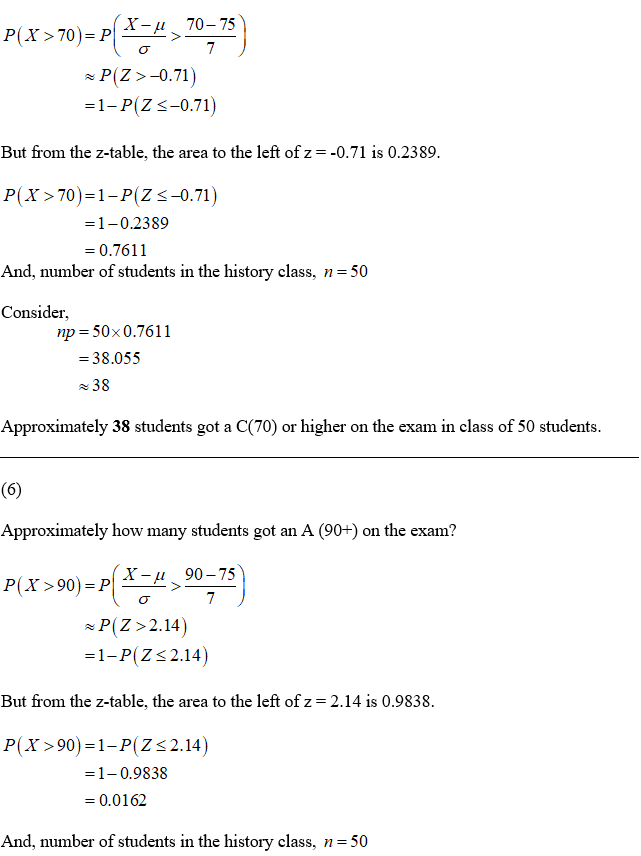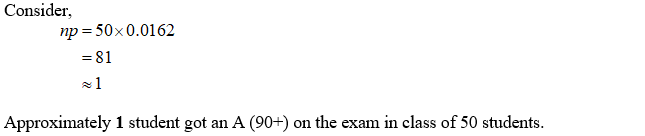## Related Solutions

##### A statistics professor gave a 5-point quiz to the 50 students in his class. Scores on...
A statistics professor gave a 5-point quiz to the 50 students in his class. Scores on the quiz could range from 0 to 5: The following frequency table resulted: (1.5 points) Quiz Score f rf cf crf c% 5 4 .08 50 1.00 100% 4 10 .20 46 .96 96% 3 14 .28 36 .72 72% 2 10 .20 22 .44 44% 1 8 .16 12 .24 24% 0 4 .08 4 .08 8% 1. Compute the values that define...
##### Suppose that student scores on creativity test are normally distributed. The mean of the test is...
Suppose that student scores on creativity test are normally distributed. The mean of the test is 150 and the standard deviation is 23. Using a z-table (normal curve table), what percentage of students have z-scores a) below 1.63 b) above -0.41 Using a z-table, what scores would be the top and bottom raw score to find the c) middle 50% of students d) middle 10% of students Using a z-table, what is the minimum raw score a student can have...
##### Suppose that student scores on math skills test are normally distributed. The mean of the test...
Suppose that student scores on math skills test are normally distributed. The mean of the test is 35 and the standard deviation is 4. Using a z-table (normal curve table), what percentage of students have z-scores a) below 2.05 b) above -0.50 Using a z-table, what scores would be the top and bottom score to find the c) middle 15% of students d) middle 25% of students Using a z-table, what is the minimum raw score a student can have...
##### The scores on the Test for the herbology course at Hogwarts were normally distributed, and the...
The scores on the Test for the herbology course at Hogwarts were normally distributed, and the z scores for some of the students are shown below: Harry: 1.10 Hermione: 1.70 Neville: -2.00 Ron: 0.00 Draco: -0.80 Luna: 1.60 If the mean score was μ=150 with standard deviation σ=20, what was the test score for each student? (matching) ___Harry. A.184 ___Hermione B.182 ___Neville   C.110 ___Ron   D.134 ___Draco E.172 ___Luna F.150
##### Suppose test scores in a nutrition class are normally distributed. What is the probability that a...
Suppose test scores in a nutrition class are normally distributed. What is the probability that a randomly selected student’s score is three standard deviations above the mean? A.) Not enough information B.) 0.9987 C.) 0.0013 D.) 0 E.) 0.0026
##### Scores on a statistics final in a large class were normally distributed with a mean of...
Scores on a statistics final in a large class were normally distributed with a mean of 72 and a standard deviation of 4 . Use the Cumulative Normal Distribution Table to answer the following. (a) Find the 37 th percentile of the scores. (b) Find the 70 th percentile of the scores. (c) The instructor wants to give an A to the students whose scores were in the top 12 % of the class. What is the minimum score needed...
##### Scores on a statistics final in a large class were normally distributed with a mean of...
Scores on a statistics final in a large class were normally distributed with a mean of 76 and a standard deviation of 9. (a) Find the 25th percentile of the scores. (b) Find the 79th percentile of the scores. (c) The instructor wants to give an A to the students whose scores were in the top 13% of the class. What is the minimum score needed to get an A? (d) Between what two values is the middle 60% of...
##### Scores on a statistics final in a large class were normally distributed with a mean of...
Scores on a statistics final in a large class were normally distributed with a mean of 71 and a standard deviation of 8. Use the Cumulative Normal Distribution Table to answer the following. (a) Find the 30th percentile of the scores. (b) Find the 70th percentile of the scores. (c) The instructor wants to give an A to the students whose scores were in the top 5 % of the class. What is the minimum score needed to get an...
##### . Suppose the scores on a chemistry test were normally distributed with a mean of 78...
. Suppose the scores on a chemistry test were normally distributed with a mean of 78 and a standard deviation of 10. If a student who completed the test is chosen at random, Find the probability that the student earned fewer than 75 points. Find the probability that the student earned at least 70 points. Find the probability that the student earned between 80 and 90 points. Find the probability that the student earned either less than 80 points or...
##### For a certain standardized placement test, it was found that the scores were normally distributed, with...
For a certain standardized placement test, it was found that the scores were normally distributed, with a mean of 190 and a standard deviation of 30. Suppose that this test is given to 1000 students. (Recall that 34% of z-scores lie between 0 and 1, 13.5% lie between 1 and 2, and 2.5% are greater than 2.) (a) How many are expected to make scores between 160 and 220? (b) How many are expected to score above 250? (c) What...